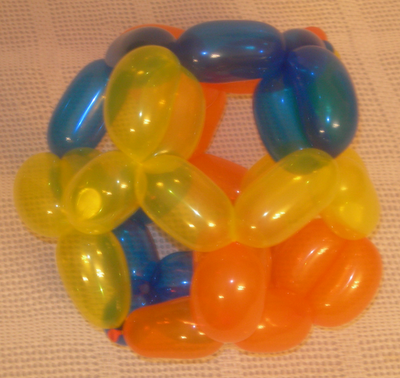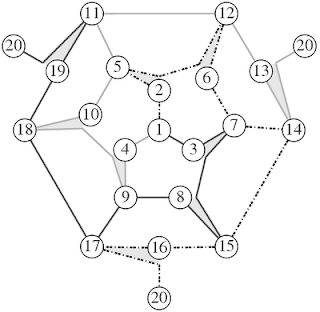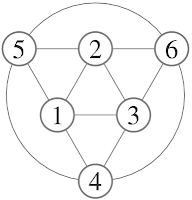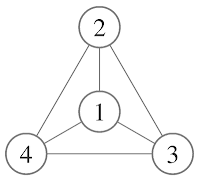Sunday, August 24, 2008

The Balloon DodecahedronThe dodecahedron is a tricky structure to make out of a small number of balloons. There are twenty degree 3 vertices and thirty edges. For symmetry, we need the number of balloons to divide the number of edges but as well figure out how to deal with the odd degree vertices. I have designed a 2-balloon dodecahedron but it was not very aesthetically pleasing. The design I currently prefer is a 3-balloon design. The trick was to instead of using all the ends of the balloons to each take care of an odd degree vertex leaving fourteen (which is not divisible by three), was to have two vertices where three ends come together leaving eighteen vertices of degree 3 (which is divisible by three).

In this design, I use nine doubled edges. The doubled edges stabilize the shape as well as locking the balloons local geometry. To make the weaving of the balloons easier, the doubling of the edges are delayed until the other balloons are in place. This delay reduces the tension on the twisting.

Below is a video of the construction of a dodecahedron.

Here is the psuedo-gauss code for this design.

1a 2 5 2d 6 12 6e 7b 14 15e 16 17 16 20
1a 3 7b 3 8 15 8e 9c 17 18e 19 11 19 20
1a 4 9c 10 18e 10 5d 11 12e 13 14 13 20

* the superscript means a delayed connection. For example in the first balloon, the first line, the sequence 2 5 2d 6 12 6e 7b can be created all at once as a string of 7 segments like a string of sausages. Even though the 2 is repeated, it is not joined until the third balloon reaches the d (see the 5d). Then the two segment 2-5 and 5-2d can be twisted together to form a double edge.The graph has a three-fold rotational symmetry (C3). To keep the symmetry of the graph, node 20 has three copies but really it is a single node at infinity.

Friday, August 22, 2008

The Balloon IcosahedronThe icosahedron is another platonic solid that has all of its vertices with odd degree. What makes it a little trickier than the cube is that it has twelve degree 5 vertices and 30 edges (instead of 8 vertices of degree 3 and 12 edges of the cube). To ease in complexity of remembering the balloon design, symmetry in the use of balloons is preferred. Essential this means that each balloon could rotated into the position of an other balloon. Symmetry dictates the number of balloons should divide the number of edges so 3 balloons is a good choice. This would have ten segments per balloon.

With three balloons, we have six ends that can take care of six of the twelve degree 5 vertices. The other six vertices with odd degree can be turned into degree 6 vertices by joining them to an interior point. The video below demonstrates the construction but perhaps the psuedo-gauss code is more explicit.From the graph of the balloon, you can see that symmetry of the balloons since you can rotate the graph 120 degrees and the shading of the lines (which represent the colour of the balloon) all become swapped. A further symmetry is that it can be inverted from start to end. This describes a D3 symmetry group.

Here is the psuedo-gauss code:

1z 3 9 10 5a 6 9c 8d 2 6 11 12z

2z 1 5a 11 7b 8d 0 5 4e 3 8 12 10z

3z 2 7b 12 9c 4e 0 7 6 1 4 10 11z

Thursday, August 21, 2008

The Balloon CubeThe cube balloon, like the tetrahedron balloon, has the problem of having odd degree vertices. By having two balloons, four of the problem vertices can be dealt with. The other four will be dealt with by going through the interior point once for each balloon turning those vertices into degree 4 vertices. To symmetrically space these degree 4 vertices, I used the vertices so that none share an edge (the points form a tetrahedron).

3 4 1 5a 0b 2 6 7 8
6 5 8 4a 0b 7 3 2 1

This design thus use two balloons with eight segments each, which allows for large segments for a large cube. Notice that the two internal segments would theoretically be 0.8660 (root 3 over 2) the length of the larger sections but I think in practice it's more like 0.7 because of the bulging properties of balloons. With only 4 internal balloons this design is very sensitive to the ratio of the external and internal balloons. This allows for some interesting patterns that are topologically equivalent but that don't look like a cube.

An alternative design that would ensure a cube look would have all 8 vertices connected to the centre point:

0z 3 4 1 5a 0b 2 6 7 8 0z
0z 6 5 8 4a 0b 7 3 2 1 0zWednesday, August 20, 2008

The Balloon OctahedronThe octahedron was the first balloon I ever made. It is by far the easiest one to make since all the vertices have degree 4. There are many one balloon paths that construct the octahedron but the one I describe here is nice because it does not require a balloon to be pulled through a triangle.

To describe this I have the following youTube videos.

Here's the original with a 350 balloon.

The psuedo-gauss code for this is

1 2 3 4 5 2 6 4 1 5 6 3 1The Balloon TetrahedronThe first balloon design is this 1-balloon tetrahedron design. It's not the easiest design but it's the easiest shape. The tetrahedron is composed of four triangles but I like to focus on the edges and vertices (I like to think in terms of graph theory). There are six edges and four vertices. The problem with the making the tetrahedron is that the vertices have degree three--this means that every vertex has a problem (see Eulerian path). A balloon comes into a vertex and leaves the vertex which takes care of two of the three edges but the remaining one has to be dealt with. So you can either have the balloon start or end at that vertex (but each balloon only has two ends) or you could cheat. There a number of ways to cheat but the one used here is to join each balloon with an interior point. With this design, each of the vertices now has degree four.

As well as a video of the construction, I'll include a diagram and a sequences of that describes the design similar to Gauss code. This is perhaps more to introduce the method of description rather than to be useful in this case. So here I'll have a planar graph with the vertices labeled but the interior point(0) omitted (which would make it non-planar).0z, 1, 2, 3, 1, 4, 2, 0, 4, 3, 0z

The z superscript indicates that the 0 is a delayed connection until the z subscript.

Saturday, August 09, 2008

Balloon polyhedraSince I haven't been making many polygon patterns I've decided to put my balloon designs up on my blog. I'll try to space them out a bit. These will hopefully end up in a paper soon. Here are the platonic balloons that I have designed. Aside from the tetrahedron, these are a little tricky to design. But for now enjoy the picture. Eventually I'll have a photographer take pictures of these. I'll post the designs soon.